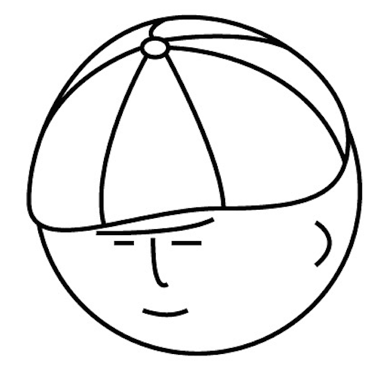# マツシタのお勉強メモ

#### マツシタのお勉強

##### welcome to my engineering blog
###### Hacker Rank# 【グラフ理論とネットワーク理論】Network and Flow #3

Introduction Here is the article I wrote last time class. keita-matsushita.hatenablog.com Network and Flow Network is defined as below. ・Network : Graph G(V, E) in which branch capacity Ci(> 0) is defined. ・f(vi, vj) : Branch flow value …

# 【グラフ理論とネットワーク理論】Matrix Expression of Graphs and Paths #2

Introduction Here is the article of the last time class. keita-matsushita.hatenablog.com Matrix Expression of Graphs and Paths Graphs can be expressed by matrixes. Below matrixes express above the graph. Incidence matrix (接続行列) Cols re…

# Find out whether there is a routes between two nodes

Problem Given a directed graph, design an algorithm to find out whether there is a route between two nodes. How to Solve This problem can be solved by depth first search or breadth first search. While traversing the graph, we have to contr…